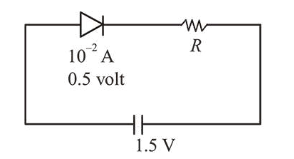# When a diode is forward biased, it has a voltage drop of 0.5 V.

Question:

When a diode is forward biased, it has a voltage drop of $0.5 \mathrm{~V}$. The safe limit of current through the diode is 10 $\mathrm{mA}$. If a battery of emf $1.5 \mathrm{~V}$ is used in the circuit, the value of minimum resistance to be connected in series with the diode so that the current does not exceed the safe limit is :

1. $300 \Omega$

2. $50 \Omega$

3. $100 \Omega$

4. $200 \Omega$

Correct Option: , 3

Solution:

(3) According to question, when diode is forward biased,

$V_{\text {diode }}=0.5 \mathrm{~V}$

Safe limit of current, $I=10 \mathrm{~mA}=10^{-2} \mathrm{~A}$

$R_{\min }=?$Voltage through resistance

$V_{R}=1.5-0.5=1 \mathrm{volt}$

$i R=1\left(=V_{R}\right)$

$\therefore R_{\min }=\frac{1}{i}=\frac{1}{10^{-2}}=100 \Omega$